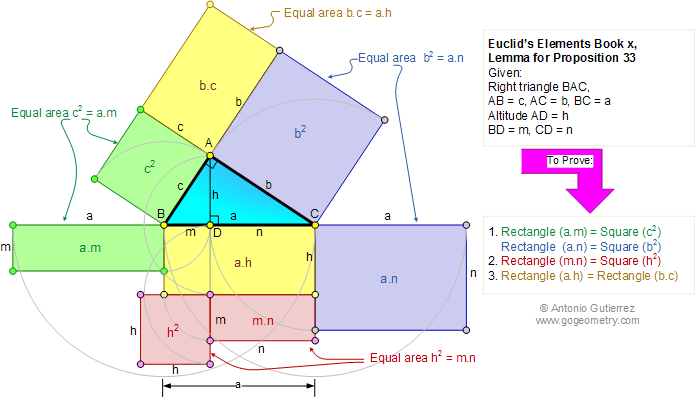Home Geometry Euclid's Elements         by Antonio Gutierrez
 Euclid's Elements Book X, Lemma for Proposition 33: Right triangle, Altitude, and Rectangles. Level: High School, SAT Prep, College geometry. Let ABC be a right-angled triangle having the angle A right, and let the perpendicular AD be drawn: Euclid says that the rectangle CB, BD is equal to the square on BA, the rectangle BC, CD equal to the square on CA, the rectangle BD, DC equal to the square on AD, and, further, the rectangle BC, AD equal to the rectangle BA, AC. Post a comment.Recent Additions